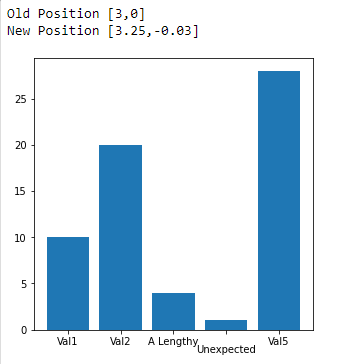# Using 'set_x' to move x-axis ticklabel

Here is an example plot along with a dataset.

``````fig, ax = plt.subplots(figsize = (5,5))
x_data = np.arange(0,5,1)
y_data = [10,20,4,1,28]
x_labels = ['Val1', 'Val2', 'A Lengthy', "Unexpected", 'Val5']
ax.bar(x_data, y_data, tick_label = x_labels)
``````I wanted to move the `Unexcpected` label a little to the right. So I thought of using this

``````for val in ax.get_xticklabels():
if val.get_text() == "Unexpected":
x,y = val.get_position()
print(f'Old Position [{x},{y}]')
val.set_y(-0.03)
val.set_x(x + 0.25)
x,y = val.get_position()
print(f'New Position [{x},{y}]')
``````

Here is the outcomeThe label moved downwards but not to the right. Is this a bug or something? I wanted to know why this isn’t working. I have found a solution using transforms here but I was hoping if `set_x` could work or to know what it’s correct usage